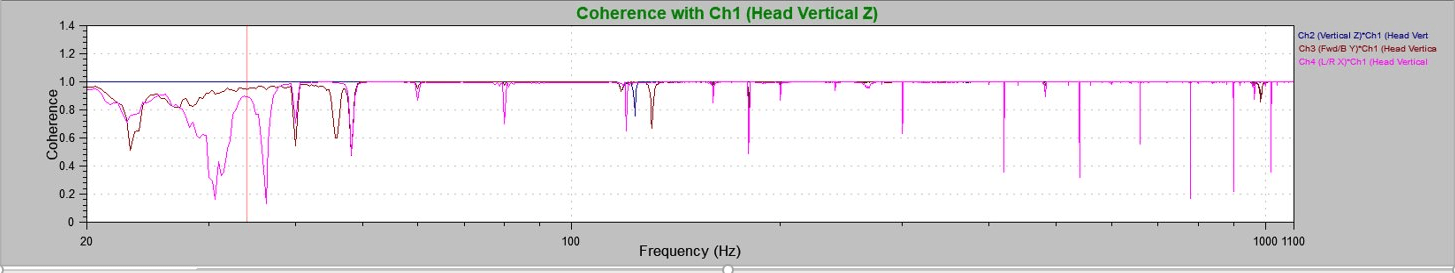# Analyzer Package

### Delivering Testing Insights using the Analyzer Software Package

Sometimes it is difficult to get clear insight into how a product is responding to a vibration test, especially if it is a complex product. Frequency-dependent interactions can occur between the vibrations of multiple components, and the interactions can move across all three axes. Basic vibration graphs, like an FFT or PSD, may hint at these interactions, but the details remain elusive.

Test engineers need efficient and reliable ways to get a deeper understanding of complex vibration relationships. Ideally, they should be able to select multiple signals of interest and then compare them: (1) efficiently, using a simple, intuitive user interface, and (2) graphically, so significant relationships between signals are readily apparent.

Fortunately, there is a tool specifically designed to give a deeper understanding of complex vibration relationships. The Analyzer Package, an optional module in the VibrationVIEW software suite, enhances the Sine, Random, and Shock test functions by comparing the characteristics of two or more signals in a series of graphs. Collected data is displayed by four advanced graphing functions, all accessible via the VibrationVIEW user interface. These graphing functions are:

• Transfer Function uses two graphs to compare:
1. the ratio of PSD values for two signals across a specific frequency range and
2. the phase relationships between the two signals.

The Transfer Function graph helps test engineers determine if two different signals are behaving in a similar manner. It is used with Random and Shock tests.

A Transmissibility graph, available as a standard VibrationVIEW feature, also shows the PSD relationships between two signals. Adding the phase relationship graph is unique to the Transfer Function.

• Total Harmonic Distortion (THD) determines the amount of harmonic content in a signal by comparing the accelerations at a specific frequency with the accelerations at the next 10 harmonics of that frequency. THD can be used as a measure of how well a shaker recreates the signal from a controller. It is used with Sine tests.
• Coherence plots the correlation calculation value across the frequency domain. Coherence is a statistic that examines the relationship between two values/signals. Two signals are coherent if they have a constant phase difference and the same frequency and waveform. Coherence graphs are used with Random and Shock tests.An example of a coherence plot of vibrations in the Y axis (ch.3) and X axis (ch.4) compared to shaker head vibrations (vertical) (ch.1). At several frequencies the X and Y axis signals do not correlate well with the vertical axis vibrations

• Cross Spectrum compares one signal with another signal in time. For example, the Cross Spectral Density may compare the drive signal with an input signal. When comparing signals from two different sources, there may be a time delay in the measurement. Consequently, there also will be a phase difference between the two signals. Therefore, Cross Spectral Density will contain data regarding both the differences in the amplitude of the two signals and the differences in the phases of the two signals. These graphs are used with Random and Shock tests.

For an in-depth review of the Analyzer Package functionality, select the link below to see our course in VRU. If you’re working with complex vibration tests, it might be what you need.

Date

April 29, 2019

Author

Thomas Roberts

Category

Analysis